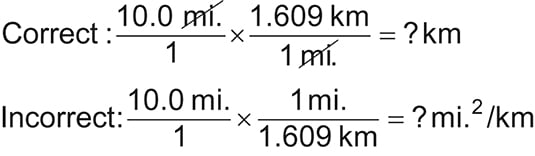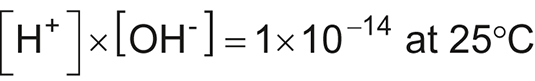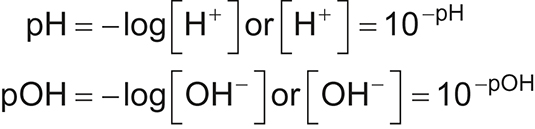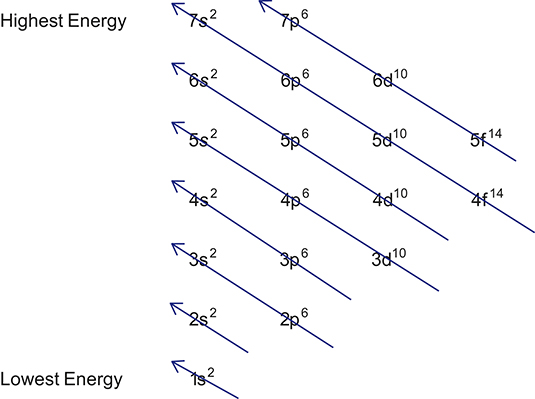##### Chemistry: 1001 Practice Problems For Dummies (+ Free Online Practice)Solving chemistry problems is a great way to master the various laws and calculations you encounter in a typical chemistry class. This Cheat Sheet provides some basic formulas, techniques, and tips you can refer to regularly to make solving chemistry problems a breeze (well, maybe not a breeze, but definitely easier).

## Keeping track of units in chemistry calculations

When completing a chemistry calculation, always write the number with the unit. Number, unit — it’s like having a first and last name.

Consistently writing down the units allows you to see what cancels and helps you spot an error when units don’t cancel. For example, look at the following conversion from miles to kilometers. The correct setup gives you an answer in kilometers. The incorrect setup gives you an answer in square miles per kilometer, which doesn’t make sense.## Balancing chemical equations

A chemical equation shows what happens in a chemical reaction. As in any equation, the elements on the left side of the equation (the reactants) must equal the elements on the right (the products). Here are just a few things to remember when balancing chemical equations:

• Same elements in equal numbers: For an equation to be balanced, it must have the same number of each element on each side of the equation. The mass of the reactants has to equal the mass of the products — remember the law of conservation of mass?

• Same charge: If there’s a net charge on one side of the equation, the other side of the equation must have the same charge.

• Unchanged chemical formulas: Most importantly, never alter a chemical formula! Subscripts (and superscripts for that matter) do not magically change. Change only the coefficients.

## Formulas for solving problems dealing with acids and bases

Acid-base reactions and their associated calculations play a primary role in many chemical, biological, and environmental systems. Whether you’re determining hydrogen ion concentration, [H+]; hydroxide ion concentration, [OH˗]; pH; or pOH, an equation and a calculator are important tools to have in your toolbox. Following are some handy formulas for solving acid/base problems.

## Calculating hydrogen or hydroxide ion concentration

The following equation allows you to calculate the hydrogen ion concentration, [H+], at 25°C if you know the hydroxide ion concentration, [OH]; you can also find [OH] if you know [H+]. Just divide 1 × 1014 by the concentration given, and you get the concentration that you need.To use scientific notation on your calculator, use the EE or EXP key (followed by the exponent) rather than the × 10^ keys.

## Calculating hydrogen or hydroxide ion concentration from the pH or pOH

Be familiar with how to solve for [H+] or [OH] when given the pH or pOH (or vice versa). Use the following formulas:Many scientific and graphing calculators differ in how they handle inputting values and taking logarithms, so know the proper keystroke order for your calculator. Be sure to review your calculator manual or look online.

## Calculating pH when given the pOH

Calculating pH when you know the pOH (or vice versa) is probably the easiest of the acid-base calculations. Here’s the formula:

pH + pOH = 14

Simply subtract the given value from 14 (keeping significant digits in mind) to get the value that you need.

## Doing titration calculations with a 1:1 acid-to-base ratio

When you’re given titration calculations where the acid and base are reacting in a 1:1 ratio according to the balanced equation, the following equation offers a quick and easy way to solve for either the concentration of one of the substances or the volume necessary to complete the titration:

MAVA = MBVB

If the acid and base aren’t reacting in a 1:1 ratio, use stoichiometry (or dimensional analysis) to solve for your unknown quantity. By the way, stoichiometry works for the 1:1 ratio questions, too; it just takes one or two more steps.

Keep track of your units! Cancel what you need to get rid of and make sure that you still have the units you need in your final answer.

## Writing electron configurations

An electron configuration is a description of the relative locations of electrons in an atom or ion. Electron configurations are based primarily on three principles: the Aufbau principle, the Pauli exclusion principle, and the Heisenberg uncertainty principle.

The most important thing to remember is that electrons fill orbitals from lowest energy to highest energy. Think about a hotel with many floors and a broken elevator; getting to the highest floor takes energy — lots of energy. Electrons conserve energy so that the lowest-energy level orbitals fill first.

You can use the following diagram, which is sometimes referred to as the diagonal rule, as an aid when writing electron configurations. Notice that putting an electron in a 3d orbital takes more energy than putting one in a 4s orbital, because 3d orbitals are more complex.

There are other ways to remember the order in which orbitals fill — you can memorize the sequence or use a periodic table.You may have to write an electron configuration for an ion instead of an atom. If you need to write a configuration for a cation (positive ion), remember to subtract the number of electrons equal to the charge from the total number of electrons before starting. Likewise, if you’re writing a configuration for an anion (negative ion), remember to add the number of electrons equal to the charge to the total number of electrons before starting.

Electron configurations only get more difficult as you choose elements with higher numbers of electrons. You can generally check your work by adding up all the superscripts to make sure that you have the right number of electrons. But note that some elements are exceptions to the diagonal rule.

Your instructor or book should mention these elements and let you know if you’re responsible for knowing how to write their configurations.